Xavier Vilà (2008)

A Model-To-Model Analysis of Bertrand Competition

Journal of Artificial Societies and Social Simulation vol. 11, no. 2 11
<https://www.jasss.org/11/2/11.html>

Received: 04-Aug-2007    Accepted: 16-Mar-2008    Published: 31-Mar-2008###Abstract

This paper studies a version of the classical Bertrand model in which consumers exhibit some strategic behavior when deciding from what seller they will buy. We use two related but different tools. Both consider a probabilistic learning (or evolutionary) mechanism, and in the two of them consumers' behavior influences the competition between the sellers. The results obtained show that, in general, developing some sort of loyalty is a good strategy for the buyers as it works in their best interest. First, we consider a learning procedure described by a deterministic dynamic system and, using strong simplifying assumptions, we can produce a description of the behavior of the process. Second, we use finite automata to represent the strategies played by the agents and an adaptive process based on genetic algorithms to simulate the stochastic process of learning. By doing so we can relax some of the strong assumptions used in the first approach and still obtain the same basic results. It is suggested that the limitations of the first approach (analytical) provide a good motivation for the second approach (Agent-Based). Indeed, although both approaches address the same problem, the use of Agent-Based computational techniques allows us to relax hypothesis and overcome the limitations of the analytical approach while obtaining the same basic results.

Keywords:
Agent-Based Computational Economics, Model-To-Model Analysis, Evolutionary Game Theory, Imperfect Competition

###Introduction

1.1
The well-know Bertrand model of duopolistic competition has somehow puzzled economists since its publication (Bertrand 1883). The main result of this model states that if two firms selling the same homogeneous product, both with identical cost functions and facing the same demand function, compete in the determination of prices, then the only equilibrium is that both firms will set prices equal to marginal cost, hence earning zero profits. Such outcome arises as the result of both firms undercutting each other's price in order to gain market share (it is assumed that consumers will buy from the firm selling at the lowest price). This result has many times been deemed as paradoxical in the sense that real competition does not seem to corroborate the prediction of the model.

1.2
Many authors have suggested that this apparent paradox might be the result of the restrictive assumptions used in the model such as, among others,
1. Static setting: The Bertrand model assumes that firms fix prices simultaneously and forever. In the real world, though, firms fix prices as a reaction to its competitor behavior in a dynamic process that involves adaption (learning) and strategic thinking.
2. Homogeneous goods: The Bertrand model assumes that the goods offered by the two firms cannot be distinguished by the consumers. Nevertheless, identical goods do not seem to exist in the real world. Commodities differentiate in brand-name (at least), location, advertising, etc.
3. Consumers do not behave strategically: The model assumes that consumers will buy from the firm selling the (homogeneous) product at the lowest price, thus discarding any possibility of strategic behavior on the demand side. But if, as in real competition, products are not totally homogeneous and competition is a dynamic process, then some attention should be paid to consumers' behavior.
4. No capacity constraints: Finally, the model assumes that if one firm sets a price strictly below its competitor's, then it gains the whole market. Again, in the real world it is hardly likely that one single firm could serve the whole market. It is clear that capacity constraints should be taken into account.

1.3
Several authors have addressed the first issue (static vs. dynamic setting) in different ways. For instance, in (Dutta, Matros and Weibull 2007) long-lived consumers are introduced to show that the longer they live, the easiest is to obtain a result similar to the one on the original model. Also, Dudey (1992) considers a repeated competition model and shows that, if capacity constraints are not binding (i.e. one single firm can serve the whole market as in the original model), then the equilibrium also corresponds to both firms earning zero profits. With regards to how to model this dynamic setting, Alchian (1950) already suggested that evolutionary models could suit well the dynamics underlying competition among firms.

1.4
Regarding the second issue (homogeneous goods vs. product differentiation), the models of differentiated Bertrand competition (monopolistic competition) are nowadays standard in the literature. Also, Deneckere, Kovenock, and Lee (1992) introduce loyal (brand aware) consumers that might not abide by the rule of buying from the cheapest seller. They show that consumer loyalty plays an important role in the determination of the price leader in the market.

1.5
The third issue (non-strategic vs. strategic buyers' behavior) has also been considered in several works. As Hehenkamp (2002) points out, the Bertrand model of duopoly relies upon two important assumptions regarding consumer's behavior: (i) consumers search at zero cost for the firm setting the lowest price and, (ii) switching firms has to be free. Nevertheless, not many papers explicitly take into account consumer's behavior when analyzing the competition among firms. Harrington and Chang (2005), and Ferdinandova (2003) analyze to what extend consumer's behavior can influence market dominance and, thus, can contribute to "shape" the competition among firms. More related to our work, Hehenkamp (2002) shows that the "sluggishness" (or not) of consumers when searching for the best price can critically influence the outcome of the classical Bertrand model, that is, depending on how consumers behave firms could, in equilibrium, set prices equal to marginal cost or higher. These three papers use different evolutionary techniques for their analysis.

1.6
Here we also present an evolutionary (and thus dynamic) analysis of firm competition in which consumers are allowed to exhibit some strategic behavior when deciding from which one of the two sellers they will buy. Moreover, as discussed below, we will use two different approaches to the problem: one analytical and another using Agent-Based Computational techniques. We will take into account not only the behavior of consumers when searching for the best price but also the role that a positive cost of switching firms might have. Finally, as opposed to the model by Hehenkamp, we will assume that the competition among firms is modeled as a repeated game which, to our understanding, fits better to the social evolutionary dynamics.

1.7
The objective of our research is twofold. On the one hand, along the lines discussed above, we want to contribute to the analysis of price competition by exploring the role played by consumer loyalty in an evolutionary setting that models the process of learning (or adaption). On the other hand, we perform a Model-to-Model analysis (Hales, Rouchier, and Edmonds 2003) of the above scenario to show how the standard, formal mathematical analysis of firm's competition can be complemented and enhanced by means of Agent-Based Computer Simulations

1.8
In this later sense, we first consider the case in which the learning procedure can be described by a deterministic dynamic system that uses expected values based on the work of Nowak et al. (2004). Using strong simplifying assumptions we are able to solve this case and to produce a complete description of how the learning process behaves. We also discuss the problems that one might face when trying to relax some of the assumptions made.

1.9
We then proceed to our computational model based on the analysis of the repeated Prisoner's Dilemma using Genetic Algorithms by Miller (1996). We use finite automata to represent the strategies played by the sellers (and also by the buyers) and a decentralized adaptive process based on the models of genetic algorithms to simulate the stochastic process of learning or evolution. With this technique we can relax some of the strong assumptions used in the first approach and still obtain the same basic results. Additionally, as a methodological agent representation issue, we follow Vilà (1997) and modify the standard operators used in genetic algorithm techniques to make them more suitable to social (or economic) simulations.

1.10
We like to think that, to some extent, the limitations of the first approach (analytical) provide a good motivation for the second approach (Agent-Based simulations). Indeed, although both approaches address the same problem, we show that the use of Agent-Based computational techniques allows us to relax hypothesis and overcome the limitations of the analytical approach while the results obtained can be easily put in contrast with those in the first approach.

1.11
The model consists of two sellers, offering the same homogeneous product, that compete against each other in a market with a given number ( m) of potential buyers. At each period t (t=1, 2, …, T) the market opens (and closes) a fixed number of times ( R) that we will refer to as rounds. It is important to notice the difference between periods and rounds. Each period (for instance, a week) contains R rounds (for instance, 7 days). The two sellers decide at the beginning of each period a strategy that specifies the course of action to take at each of its rounds contingent on what happened in previous rounds of the same period. Thus, at the beginning of each round, the two sellers set a price for their product according to their strategies for that period. Then, the market opens and the two prices become known to everybody. The m buyers walk in and each of them decides from which of the two sellers will buy based on the observation of the two prices. Trade takes place and a new round begins. After R rounds (end of the period) both sellers update their per period strategies according to some procedure that will be specified.

1.12
The idea behind this sequence of events is that each seller uses the R rounds to learn about the behavior of its competitor. Thus, each period represents a step in a learning procedure. The model can also be understood as an evolutionary model where each period represents the end of an old generation of sellers and buyers and the birth of a new generation that inherits the characteristics of its parent generation with, hopefully, some improvements. We will refer to the two intuitions indistinctly.

1.13
As said before, we will approach the resolution of the situation sketched above using two related but different tools (Model-to-Model Analysis). The two of them consider a probabilistic learning (or evolutionary) mechanism and in the two of them we will investigate to what extent consumers' behavior can affect the competition between the sellers.

###The deterministic system

2.1
In order to simplify the analysis and to avoid the interference that different demand functions could produce in our model, we assume that, at each period t, the sellers can only choose between two possible prices, namely a high price ph and a low one pl. This is, indeed, a very strong simplification, although Hehenkamp and Leininger (1999) argue that from an evolutionary modeling perspective it makes sense to consider a discrete list of possible prices only. The Agent-Based approach in section 3 could easily overcome this limitation, but then we would loose the reference of the analytical approach for comparisons. We further assume that at the beginning of each period the set of strategies available to the sellers consists only of the following:
• Always cooperate (C), which means that the seller using this strategy will always set a high price ph -and thus will "cooperate" with its competitor- regardless what happened in previous rounds of the current period.
• Always defect (D), which means that the seller using this strategy will always set a low price pl -and thus will "defect"- regardless what happened in previous rounds of the current period.
• Tit for Tat (T), which means that the seller using this strategy will start each period with a high price ph and then, at each successive round in that period, will mimic what its competitor did in the previous round.
Again, the assumption that only three possible strategies are available to the sellers is a very restrictive hypothesis. Nevertheless, by adopting it we can build on the analysis by Nowak et al. (2004) and apply it to our case. We will later discuss on the essential difficulties of dropping this assumption.

2.2
Without loss of generality, we can normalize the number of buyers so that m = 2. To begin with, we assign to the high and low prices the values ph = 3 and pl = 2.We will first assume that the consumers' behavior is fixed so that they will always buy from the cheapest seller and, in the case that the two of them set the same price, they will split equally. Matrix A summarizes the per period payoff for each seller in this situation. The first row of the matrix specifies the payoff that a C-strategist receives if its opponent is a C-strategist, D-strategist, or T-strategist respectively. Similarly, the second and third rows correspond to the payoffs for a D-strategist and a T-strategist respectively. So, if a D-strategist meets another D-strategist each one receives a payoff of 2R after R rounds (one period) whereas if a D-strategist meets a T-strategist, the D-strategist gets 4 in the first round (all the buyers go to the D-strategist) and 2 for the rest of the period.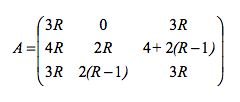2.3
Each seller, at the beginning of period t, chooses the strategy s (s∈{C, D, T}) with probability pt(s). From an evolutionary point of view, pt(s) represents the proportion of s-strategists in the total population.

2.4
Let pt be the vector of probabilities of choosing each strategy and let ptT denote its transpose.2.5
The following equation drives the dynamics of the learning/evolutionary process:where As denotes the row of A corresponding to the strategy s.

2.6
Using the learning intuition, the probability of choosing a particular strategy s is updated according to its relative performance. Indeed, Aspt represents the expected payoff of the strategy s whereas ptT·A·pt represents the expected average payoff.

2.7
From an evolutionary point of view, the dynamic equation corresponds to the dynamic system known as replicator dynamics. According to it, each strategy is replicated as a function of its relative performance with respect to the others.

2.8
In order to explore the behavior of this system we study the corresponding vector field, which is shown in Figure 1. Each point represents a probability vector pt that specifies with what probability each of the three strategies will be chosen. At each point the arrow indicates the instantaneous direction in which these probabilities evolve.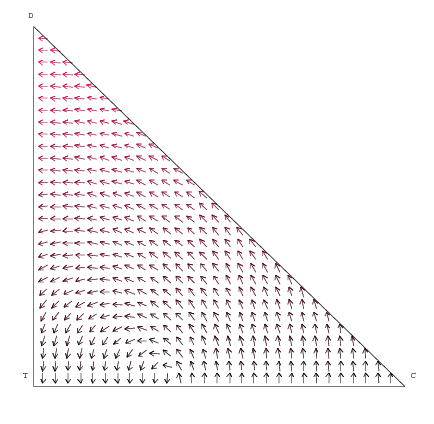Figure 1. Vector Field with non-loyal consumers

2.9
The strategy D is asymptotically stable in the sense that if pt is pushed slightly away from D by a small perturbation, it will eventually go back to D. In other words, D is evolutionary stable (Maynard Smith 1982). That is not the case for T. If pt is pushed away from T it will then converge to some point near T in the line TC but not necessarily to T again. Any point in the line TC is a rest point (although not stable) of the system since in the absence of D-strategists, C and T do equally well.

2.10
Next we assume a different behavior for the consumers and consider the case in which they are "loyal". They will still buy from the cheapest seller as in the previous case, the difference now is that in the case that the two prices are set equal, they will go back to the seller they bought from in the previous round (instead of choosing randomly). By doing so, the payoff matrix A changes since, obviously, now the first round becomes much more important as it will determine the market share of each seller. Figure 2 shows the vector field corresponding to this case. Now, any path starting in the interior of the simplex converges to D, which turns out to be the only stable equilibrium. The points in the line TC are stationary (rest points of the system) but not stable.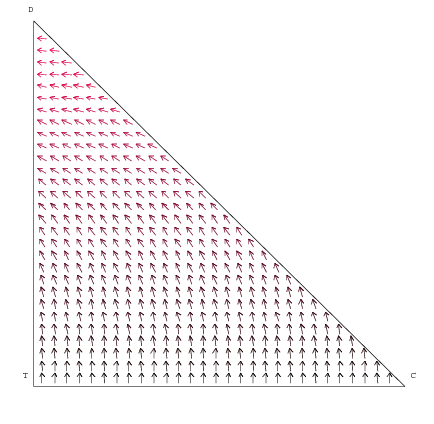Figure 2. Vector Field with loyal consumers

2.11
The change with respect to the first case is not surprising as now the competition for market share in the first round becomes critical. One might argue that in this case we should not consider the Tit for Tat strategy (begin with a high price and then imitate your competitor) since it is not a good strategy in the competition for market share. If a seller starts out by setting a high price, chances are that he will not have any customer in the next round. If we consider the opposite strategy Tat for Tit (Ta) instead, that is, start with a low price and keep it low if the competitor does so, we obtain the results depicted in Figure 3. Basically the result is the same as in the previous case in the sense that firms end up setting a low price. The difference is that in this case all the points in the line DTa are stationary but not stable (not even D).Figure 3. Vector Field with loyal consumers (and Tat for Tit)

2.12
Next we consider different values for the high and low prices. Figures 4, 5, and 6 are the equivalent to Figures 1, 2, and 3 respectively with the difference that now the high and low prices are ph = 5 and pl = 2. The size of the gap between the two prices is important since now it might not be optimal for the sellers to retaliate in order to gain market share if it requires lowering the price by more than a half. That would not make sense, for instance, if all that you expect to gain is to double your market share. The direction of the arrows in Figures 4, 5, and 6 seem to corroborate this intuition. In the first one, corresponding to the case in which consumers are not "loyal", it seems that unless there is a high probability of ruining into a D-strategist as a competitor, the mechanism is going to push the probabilities to some point in the line TC.

2.13
If consumers are loyal (Figure 5) the result is similar. It seems that we need the probability of meeting a D-strategist to be more than one half in order to converge to the low price equilibrium. In both cases, though, D continues being the only stable strategy.

2.14
If we consider Tat for Tit instead of Tit for Tat (Figure 6) we obtain very similar results to the ones in Figure 3. This is important since now it seems that being a T-strategist is not so bad after all (according to Figure 5) due to the bigger price differential and, therefore, maybe we should not substitute T by Ta in this case.Figure 4. Vector Field with non-loyal consumers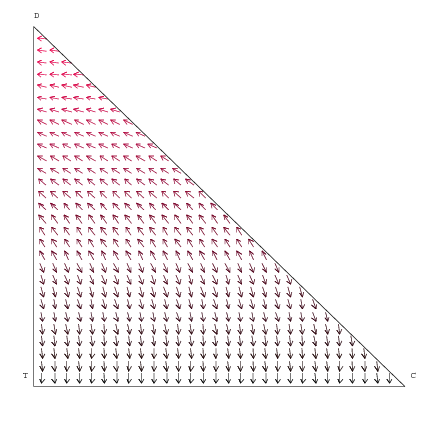Figure 5. Vector Field with loyal consumers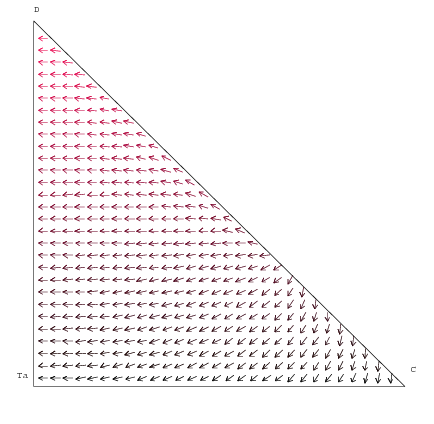Figure 6. Vector Field with loyal consumers (and Tat for Tit)

2.15
In the light of these results one might argue that it makes sense for the consumers to be loyal (at least in the very weak sense used in this model) because by doing so the sellers behave in such a way that competition works in the best interest of the consumers, that is, they pay low prices.

2.16
Even though this intuition can be thought as a nice complement to the static model of Bertrand competition, we think that the model proposed has (at least) two important limitations that make us consider a different approach. These two limitations are:
• In the model we only consider three strategies at a time. One might argue in favor of this that C, D, and T are the strategies that seem more likely to emerge from some learning or evolutionary process and, therefore, there is no need to consider more strategies since they will not be played as we approach to a limit solution. This reasoning, though, does not always work. We have an example of that in Figures 2 and 5. According to Figure 2, Tit for Tat is not a good strategy, but it turns out to be good when we consider a different set of prices. Thus, a strategy can turn out to perform relatively well or not depending on the particular set up that we have. Since we do not know before hand (if we knew we would not need to solve the problem), we do not have any reason a priori to include a particular strategy and discard another.
• Another limitation is that the interpretation that consumers will be "loyal" because if they do then competition will "evolve" in their best interest involves a strong rationality assumption. Indeed, we are not only assuming that consumers are rational maximizers perfectly informed but also that they are able to understand how evolution works so that they can predict and even control the path of evolution. We should, somehow, endogenize consumers' behavior into the system so that the agents (both sellers and buyers) learn (or evolve) simultaneously.

2.17
On the other hand, it is easy to recognize the difficulties involved in relaxing any of these assumptions. Imagine that we want to expand our set of three strategies. The question now is what additional strategies we want to consider and why. Choosing an additional strategy can be as arbitrary as not choosing it. One might use some criteria to guide this decision. For instance, a good criterion would be: "consider only strategies according to which the action taken by one of the players depends only on his past move and on the past move of his rival". The problem now is that there are 26 different such strategies. It is clear that now we cannot use the same technique since it would imply having to work with vector fields in a 25-dimensional simplex. In fact, working with more than 3 strategies is already a challenge.

2.18
Next we propose an Agent-Based model of adaptive learning (or evolution) based on genetic algorithm techniques to try to overcome these limitations. The main objective is to check if the simulation of an evolutionary process that resembles the analytical model studied in this section, but that avoids its limitations (namely a small number of strategies for the sellers and the exogenous behavior of the buyers), is able to produce comparable results. The choice of genetic algorithms as the driving mechanism of our evolutionary simulation is not arbitrary. On one hand, it is a well known and thoroughly analyzed technique and, thus, can be easily replicated, studied, and modified. On the other hand, as it will be argued in the next section, furnishing a standard genetic algorithm with a conveniently modified crossover operator makes it suitable as an "emulator" of a process of social learning by imitation.

###The Agent-Based Model

3.1
In our Agent-Based system, strategies for the repeated game will be represented by finite automata as in (Miller 1996). A finite automaton is a system that reacts to discrete inputs producing discrete outputs. Formally, a finite automaton -or Moore machine- is described by a five-tuple {Ω, Q, q0, λ, δ}, where:
• Ω is the alphabet (actions) the automata understands (Ω = {ph, pl} in our case)
• Q = {q1,…,qn}is a finite set of internal states
• q0Q is the initial state
• λ:QQ is the output function that assigns an action to each internal state
• δ:Q×Ω→Q is the transition function which maps the current internal state and the input Ω that the automaton gets from the environment to a new internal state.

3.2
For instance, the automaton in Figure 7 represents the Tit for Tat strategy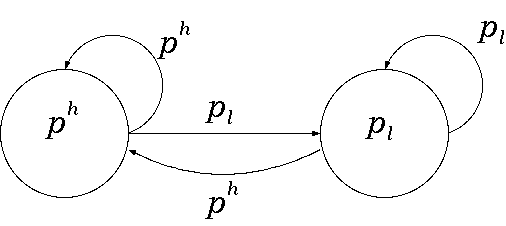Figure 7. The Tit for Tat automaton

3.3
According to this strategy, a firm will start in state 0 (initial state, left circle here) by setting a high price (ph), and will remain in that state for as long as its opponent also chooses a high price ph. If the opponent switches to a low price (pl), then the firm following the Tit for Tat automaton in Figure 7 will move to state 1 (right circle) and will set a low price in response. Then the firm will remain in this state unless its opponent switches back to setting a high price.

3.4
Following this model, the strategies for the sellers will be represented by automata of size 2 (two internal states, labeled 0 and 1). With this we restrict ourselves to consider only 26 different strategies since only 26 different strategies can be represented by finite automata of size 2. Binmore and Samuelson (1992) provide the list of such strategies for the case of the Repeated Prisoners' Dilemma. These automata can be represented by a binary string of 7 bits according to the following scheme:
• First bit : Initial state (0 or 1) that indicates what is the price to set in the first round of each period.
• Second bit : Action when at state 0. 0 means that the low price pl is chosen and 1 corresponds to the high price ph.
• Third bit : Transition state if the rival sets a low price pl. (0 or 1)
• Fourth bit : Transition state if the rival sets a high price ph. (0 or 1)
• Fifth, sixth and seventh bits : Equivalent to second, third and fourth bits but corresponding to state 1.

3.5
Thus, the automaton 0 1 1 0 0 1 0 represents a Tit for Tat strategy as in Figure 7.

3.6
One of the novelties of this model with respect to the deterministic model discussed in section 1 is that now the buyers will also be represented by finite automata (of size four) that will evolve jointly with the global evolution of the system. This is a much realistic assumption rather than taking their behavior as given. These automata representing the buyers have 4 internal states (while the sellers' automata only have 2 states) because buyers' strategies are more involved. Indeed, they have to specify whether a buyer should remain loyal (or switch to the other firm) depending on the price set by its current firm is higher, lower, or equal to the price set by the competitor. In this sense, a buyer's strategy is represented by a binary string of 30 bits corresponding to the following structure:
• The first two bits form a number that specifies the initial state (0, 1, 2, or 3)
• The rest of the bits are structured in 4 groups of 7 bits. Each of these groups corresponds to an internal state. In each of these internal states:
• the first bit corresponds to the action to take at that state. 0 means that the buyer using this strategy will buy from the same seller than in the previous round (loyalty) and 1 corresponds to the buyer switching to the other seller.
• The six remaining bits correspond to the transitions states (expressed in base 2) depending on the price paid by the buyer in the market being higher, equal or lower (respectively) than the price set by the other seller.

3.7
The evolutionary nature of the system will be driven by an adaptive mechanism based on a modified genetic algorithm that can be outlined as follows:
• Two initial populations, one of 32 strategies for the sellers, and another of 1000 strategies for the buyers are randomly formed. These figures are arbitrary and are chosen in conjunction with the specification of the payoff functions of the agents to determine the range of values of those payoffs.
• Randomly match each strategy of the first population (sellers) to another strategy in the same population.
• Bring each of these pairs to a simulated market in which each strategy will receive some payoff si(t) according to a market mechanism that we will describe later on.
• Form a new population of strategies for the sellers as follows:
• Include the top (in terms of payoff) 16 strategies from the previous population.
• Create 16 new strategies:
1. Randomly select two "parents" from the previous population. The probability of strategy j being selected as a "parent" is: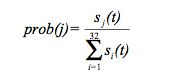2. Form two new strategies applying the crossover operator to the two parents.
3. Apply the mutation operator to the newly created strategies.
4. Repeat a, b, and c 7 times so that 14 more strategies are created.

3.8
As we have mentioned above, a market mechanism is simulated to test the performance of both the strategies of the sellers and of the buyers as well. This market works as follows:
• Two (randomly selected) sellers constitute a market that opens 5 times (5 rounds).
• At the beginning of each round, 1000 consumers walk in the market and observe the prices announced by each seller.
• Based on these prices, each consumer decides from which one will buy
• Trade takes place and each seller and buyer receive their payoffs for period t according to the following scheme:
• For the sellers: Let di,r(t) denote the number of consumers that buy from seller i at round r and let pi,r(t) the price set by seller i at round r. Then, seller's i payoff is given by• For the buyers: Let bj(t) denote the payoff obtained by strategy j belonging to generation t. Let kj(t) be the number of times that strategy j pays a low price. Then,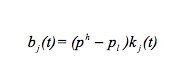3.9
In the same way as we do with the strategies for the sellers, the payoffs obtained for the strategies for the buyers are used to create a new population of strategies following a procedure parallel to the depicted for the sellers.

3.10
We perform several simulations of the procedure outlined above varying some of the parameters of the model. More specifically, we consider 9 scenarios corresponding to the following:
• Case 3A: ph = 3, pl = 2, c = 0
• Case 3B: ph = 3, pl = 2, c = 1
• Case 3C: ph = 3, pl = 2, c = 1.5
• Case 4A: ph = 4, pl = 2, c = 0
• Case 4B: ph = 4, pl = 2, c = 2
• Case 4C: ph = 4, pl = 2, c = 3
• Case 5A: ph = 5, pl = 2, c = 0
• Case 5B: ph = 5, pl = 2, c = 3
• Case 5C: ph = 5, pl = 2, c = 4.5

3.11
In all these cases, c represents a switching cost for the consumers. This parameter did not appear in the model of the previous section and, therefore, only Cases *A can be used for comparison between the analytical and the Agent-Based models.

3.12
The values for the rest of the parameters were:
• Size of populations = 32 strategies for sellers and 1000 strategies for buyers
• Number of generations (iterations) = 500
• Rounds played by each generation = 5
• Probability of mutation = 0.001
• Crossover operator = Partial imitation

3.13
In Vilà (1997) we discuss the problems generated by the classical mutation and single-cut crossover operators used in canonical genetic algorithms when applied to the above representation of finite automata. First, we use a modified mutation operator in which mutations do not take place on a locus-wise basis of the binarily encoded automata but on a state-wise basis of the underlying automata. The reason for this is that locus-wise mutations induce a non-uniform distribution over the set of automata that can be the result of the mutation of a given automata. As a consequence of this, when an automaton mutates, some automata are more likely to be the resulting "mutant" than others, which could produce an unwanted biased behavior of the genetic algorithm dynamics. Second, we modify the standard single-cut crossover operator to furnish it with a social interaction meaning. In this sense, we introduce the partial imitation crossover operator according to which two parent strings (i.e. two parent automata) can only exchange information that makes sense to exchange. This is important due to the special characteristics of the binary representation of finite automata. Indeed, it can be easily showed that the single-cut crossover operator could produce, from two identical "parents", two automata whose behavior is exactly the opposite from the behavior of their "parents". This problem can be easily illustrated by the following example:

3.14
Consider the following two automata of size 2:
 Automata 1: 0 0 0 0 1 1 1 Automata 2: 1 1 0 0 0 1 1
It is clear that the two automata represent the same strategy (but with a different representation), namely Always set a low price. Suppose now that the crossover cut point happens to be right after the first bit. What we get after performing single-cut crossover is:
 Automata 1: 0 1 0 0 0 1 1 Automata 2: 1 0 0 0 1 1 1
which corresponds to two automata representing the same strategy: Always set a high price.

3.15
This is not only awkward, but could also produce a strange behavior in the underlying dynamics. More specifically, the partial imitation crossover operator works as follows:
1. Randomly generate a fictitious history of moves by a virtual opponent of a given length. For instance: 0010.
2. Determine the move that each of the two parents would make given the sequence of inputs described by the history generated.
3. Form two new automata that reproduce the two parents but "switching" the move reported in step 2. Hence, the first new automaton will use the move reported by the second automaton if the sequence of inputs it gets is the one described by the history considered and vice versa.
It is clear that from 2 identical parents (regardless of their representation) this partial imitation operator will produce two identical automata (except for their internal structure).

3.16
Furthermore, this operator reduces significantly the number of automata that may result of the crossover of any two parents. Indeed, since the operator can only modify the bit corresponding to the action to take at a particular state we have that, from 2 automata of size n, there are 2n possible descendants at most. This limits, therefore, the importance of the specific fictitious history (randomly) chosen towards the final result of the process.

3.17
The following example illustrates how this partial imitation crossover operates on two different strategies: Tit for Tat and Always low price
 Tit for Tat: 0 1 1 0 0 1 0 Always low price: 1 0 0 0 1 1 1
Notice first that, regardless of the fictitious history generated, the Always low price strategy will always report a "0" when asked in step 2 of the partial imitation procedure above. On the other hand, the Tit for Tat strategy will report "0" in half of the histories and "1" in the other half. Hence, in 50 % of the cases, "0" will be reported by the two parent automata and thus they will produce one new Tit for Tat and one new Always Low as descendants. In the other 50% of the cases, "0" will be reported by the Always Low automata whereas the Tit for Tat automata will report "1". In these cases, the two descendants will replicate their parents by exchanging the "0" with the "1", resulting in one Always High (the Always Low automata that switches from "0" to "1") and one Always Low (the Tit for Tat automata after witching from "1" to "0" in its initial state).

3.18
It should be remarked that the above result does not depend on the particular representation of the strategy using binarily encoded automata. That is, if instead of using the strings above to represent the strategies we had used
 Tit for Tat: 1 0 0 1 1 0 1 Always low price: 0 1 0 0 0 1 1
the possible outcomes of the partial imitation crossover would be exactly the same.

3.19
To our understanding, this partial imitation operator not only solves the problem of the multiple representations of strategies by means of finite automata. Furthermore, it appears to be more similar to a process of social learning by imitation (two strategies learn from each other what to do in a particular situation following a particular sequence of events) than the standard single-cut crossover operator.

###Results

4.1
The results obtained by the final generations of buyers and sellers seem to corroborate those outlined in section 2 (see the evolution of the average payoffs in Figure 8 at the end of the paper). The important difference is that what was an assumption before is now a result, namely that in the case that the two sellers set the same price, the consumers will remain loyal to their previous seller. We have also introduced the possibility for the consumer of having an ex-ante switching cost.

4.2
A brief summary of the results obtained follows:
• Cases *A: When there are no switching costs (c = 0), the buyers will buy from the cheapest seller and will stay with their current ones if prices are equal (loyalty strategy). The sellers will set a low price regardless the price set by the opponent in the previous round (always defect strategy, D). The only difference is when the low price is too low compared to the high price (ph = 5, pl = 2) that the sellers are better off by charging always the high price. (Case 5A). Notice that these cases (when c = 0) correspond to the settings in the analysis conducted in section 2 (the deterministic system). In this sense, if we compare the results just described with those outlined in Figure 2 (for ph = 3, pl = 2 and loyal consumers) and Figure 5 (for ph = 5, pl = 2 and loyal consumers) we observe that the final pattern of behavior of the firms is basically the same in both analysis.
• Cases *B: When the switching cost equals the price difference (c = ph - pl) the buyers will buy from the cheapest seller and will stay with their current ones if prices are equal (loyalty strategy). The sellers will set a low price regardless the price set by the opponent in the previous round (always defect strategy, D). In this case, the fact that consumers bear a switching cost equal to the difference between the two prices does not make them "more loyal" in the sense of making them willing to stick to a seller (even when he sets a high price) given that there is no possible gain by switching to another. They do not want to do so because then the sellers would have some sort of monopolistic power as the next case indicates.
• Cases *C: When the switching cost exceeds the price difference (c > ph - pl) the buyers will never switch, not even in the case that someone else offers a better price. Then, sellers take advantage of this monopolistic power and always set a high price (always cooperate strategy, C).

4.3
As we look at the charts describing the evolution of the average payoffs, we observe that the convergence reached is very robust and is obtained very rapidly. Indeed, in all cases, once the average payoff reaches a steady state it remains there except for very minor fluctuations. This is consistent when the simulations are repeated several times with the same parameter values.

###Conclusions

5.1
We have analyzed the classical Bertrand model of (repeated) imperfect competition using two different but related approaches. The two of them share a common learning (or evolutive) spirit and consider the strategic behavior of the sellers and also of the buyers. The first one is based on the analysis of Nowak et al. (2004) and uses standard analytical tools to approach the model. It is shown, by using strong simplifying assumptions, that the consumers are better off by developing a "loyal" strategy since in such case the learning procedure of the buyers results in lower prices. The second approach consists of an Agent-Based model based on Miller (1996) that uses genetic algorithms to simulate the joint evolution of the behavior of both sellers and buyers. Although this approach allows for a much higher flexibility with regard to the number of parameters to consider and also to the plausibility of the assumptions made, we find that the results obtained coincide almost completely with the predictions of the analytical model.

5.2
We believe that putting these two different approaches side-by-side serves to illustrate how the use of Agent-Based computational models can help in the analysis of problems that otherwise would require strong unrealistic assumptions, or that would be difficult to solve using other techniques. Additionally, the computational model has the appealing of the evolutionary reasoning in the sense that there is no need for making strong assumptions about rational players that know and fully understand the underlying game that takes place in the market. Also, the computational model allows extending the analytical one in the sense that, due to its flexibility, one can easily introduce more parameters and variables in the analysis. For instance, if the switching cost parameter c introduced in the computational model were considered in the analytical one, its study would have been much more difficult. But the important role that this parameter plays in the computational model, for instance in Cases *B, when the consumers are willing to bear this switching cost in order to avoid getting caught in the hands of one firm, suggests that this might be an interesting issue to study in an analytical model. Hence, some findings of computational models could stimulate other approaches, thus the importance of Model-to-Model Analysis.

5.3
The Agent-Based model used, though, also has its limitations. The fact that only two prices are possible is a very unrealistic assumption. Also, the number of buyers is fixed, so that it does not matter what the prices are, the number of buyers never goes up or down. Ideally, we would like to have different types of buyers with different reservation values that would allow for a wider range of prices. Furthermore, the fact that the number of rounds R that the firms use to "learn" is fixed is another unrealistic simplification. Intuitively, the more time you spend learning, the better you learn and, hence, the resulting behavior might depend on it. Finally, another important feature of market dynamics, namely entry and exit of firms, is missing in our analysis. We believe, though, that these limitations could easily be avoided in more ambitious computational settings, and thus approach the analysis of complex imperfect competition models that would otherwise be hard to analyze using analytical techniques.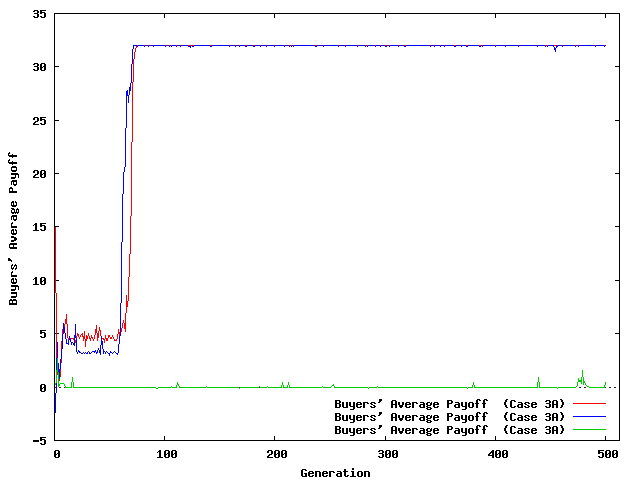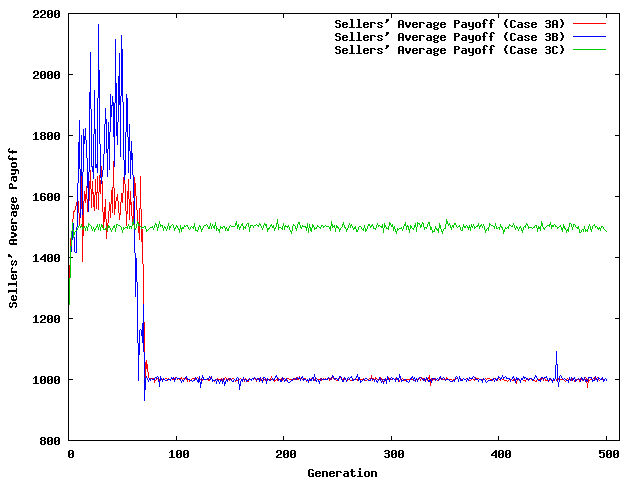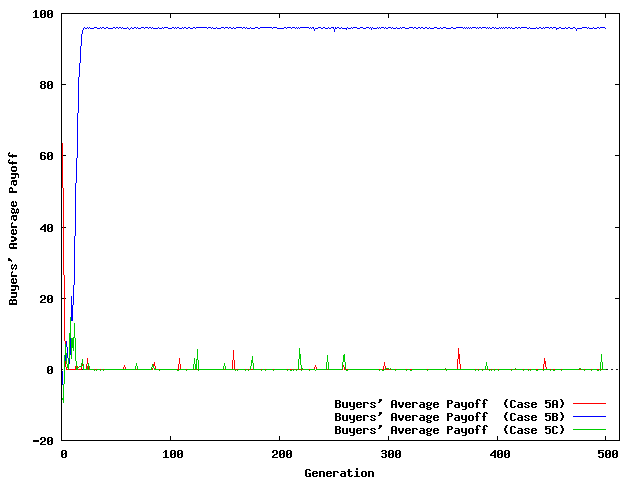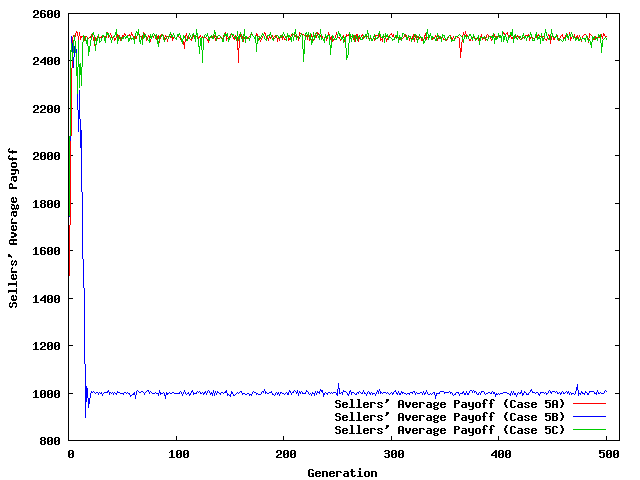Figure 8. Evolution of Average Payoffs

###Acknowledgements

Financial support from the Spanish Ministry of Education and Science through grant SEJ2005-01481/ECON and FEDER, SGR2005-0712 of the Direcció General de Recerca (Generalitat of Catalonia), CONSOLIDER-INGENIO 2010 (CSD2006-00016), and CREA (Barcelona Economics) are gratefully acknowledged.

###Notes

1 This and the mutation operator will be explained later.

###References

ALCHIAN, A. (1950). Uncertainty, evolution and economic theory. Journal of Political Economy, 58. pp. 211-221.

BINMORE, K. and Samuelson, L. (1992). Evolutionary Stability in Repeated Games Played by Finite Automata. Journal of Economic Theory, 57(2). pp. 278-305.

BERTRAND, J. (1883). Book review of theorie mathematique de la richesse sociale and of recherches sur les principles mathematiques de la theorie des richesses. Journal de Savants, 67. pp. 499-508.

DENECKERE, R., Kovenock, D., and Lee, R. (1992). A Model of Price Leadership Based on Consumer Loyalty. Journal of Industrial Economics, 40(2). pp. 147-56.

DUDEY, M. (1992). Dynamic Edgeworth-Bertrand Competition. The Quarterly Journal of Economics, 107(4). pp. 1461-1477.

DUTTA, P., Matros, A., and Weibull, J.W. (2007). Long-Run Price Competition. RAND Journal of Economics, 38(2). pp. 291-313.

FERDINANDOVA, I. (2003). On the Influence of Consumers' Habits on Market Structure. Mimeo. Universitat Autònoma de Barcelona.

HALES, D., Rouchier, J, and Edmonds, B. (2003). Model-to-Model Analysis. Journal of Artificial Societies and Social Simulation , 6(4)5. https://www.jasss.org/6/4/5.html

HARRINGTON, J. E. and Chang, M.H. (2005). Co-Evolution of Firms and Consumers and the Implications for Market Dominance. Journal of Economic Dynamics and Control, 29(1-2). pp. 245-276.

HEHENKAMP, B. (2002). Sluggish Consumers: An Evolutionary Solution to the Bertrand Paradox. Games and Economic Behavior, 40. pp. 44-76.

HEHENKAMP, B. and Leininger, W. (1999). A note on evolutionary stability of Bertrand equilibrium. Journal of Evolutionary Economics, 9. pp. 367-371.

MAYNARD SMITH, J. (1982). Evolution and the Theory of Games. Cambridge University Press, Cambridge.

MILLER, J. H. (1996). The coevolution of automata in the repeated Prisoner's Dilemma. Journal of Economic Behavior and Organization, 29(1). pp. 87-112.

NOWAK, M. A., Sasaki, A., Taylor, C., and Fudenberg, D. (2004). Emergence of cooperation and evolutionary stability in finite populations. Nature, 428(6983). pp. 646 - 650.

VILÀ, X. (1997). "Adaptive Artificial Agents Play a Finitely Repeated Discrete Principal-Agent Game". In Conte, R., Hegselmann, R., and Terna, P., (Eds.). Simulating Social Phenomena, volume 456 of Lecture Notes in Economics and Mathematical Systems, Springer-Verlag. pp. 437-456.